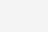# python插入排序的优化1、插入排序算法总是从有序区间搜索插入位置，以此为切入点。

2、可以使用二分搜索方法快速确认待插入的位置，所以有一个优化版本的插入排序算法，也叫二分查找插入算法。

```def insert_sort2(data_list):
'''
使用二分查找函数确定待插入元素在有序区间的插入位置
'''
count=0 #统计循环次数
length = len(data_list)
for i in range(1,length ): #默认第一个位置的元素是已排序区间，因此下标从 1 开始
print(data_list)
wait_insert_data = data_list[i] ##等待插入元素
move_index = i
insert_index,count1 = binary_search(data_list[0:i],wait_insert_data) #寻找插入位置
count+=count1 #统计循环次数需要加上二分查找的循环次数
while move_index > insert_index: #移动元素，直到待插入位置处
count+=1
data_list[move_index] = data_list[move_index - 1]
move_index -= 1
data_list[insert_index] = wait_insert_data #插入操作
print(data_list)
print(f"总循环次数为 {count}")
return data_list

def binary_search(data_list,data):
"""
输入:有序列表，和待查找的数据data
输出：data 应该在该有序列表的插入位置
count 变量纯粹是为了统计循环次数而使用的，实际应用时可去除。
"""
count = 0
length = len(data_list)
low = 0
high = length-1
##如果给定元素大于等于最后一个元素，则插入最后元素位置的后面
##如果小于第一个元素，则插入位置0
if data >= data_list [length -1]: return length,0
elif data < data_list : return 0,0
insert_index = 0
while low < high-1:
count +=1
mid = (low + high)//2 #python中的除法结果默认为浮点数取整数部分时使用 //
if data_list[mid] > data:
high = mid
insert_index = high
else:
low = mid
insert_index = low+1  #如果值相同或者值大于mid的值，那么插入位置位于其后面
return insert_index,count```